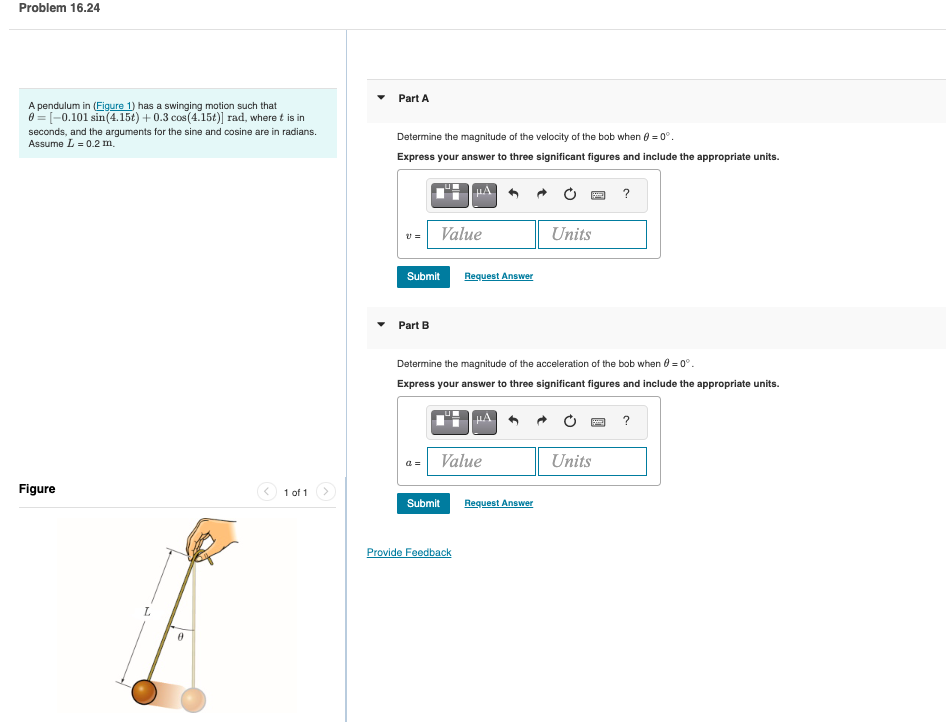# Question A pendulum in (Figure 1) has a swinging motion such that Part A $$\theta=[-0.101 \sin (4.15 t)+0.3 \cos (4.15 t)]$$ rad, where $$t$$ is in seconds, and the arguments for the sine and cosine are in radians. Determine the magnitude of the velocity of the bob when $$\theta=0^{\circ}$$. Assume $$L=0.2 \mathrm{~m}$$. Express your answer to three A pendulum in (Figure 1) has a swinging motion such that Part A θ=[−0.101sin(4.15t)+0.3cos(4.15t)] rad, where t is in seconds, and the arguments for the sine and cosine are in radians. Determine the magnitude of the velocity of the bob when θ=0∘. Assume L=0.2 m. Express your answer to three significant figures and include the appropriate units. Part B Determine the magnitude of the acceleration of the bob when θ=0∘. Express your answer to three significant figures and include the appropriate units.EAU0RT The Asker · Mechanical EngineeringTranscribed Image Text: A pendulum in (Figure 1) has a swinging motion such that Part A θ=[−0.101sin(4.15t)+0.3cos(4.15t)] rad, where t is in seconds, and the arguments for the sine and cosine are in radians. Determine the magnitude of the velocity of the bob when θ=0∘. Assume L=0.2 m. Express your answer to three significant figures and include the appropriate units. Part B Determine the magnitude of the acceleration of the bob when θ=0∘. Express your answer to three significant figures and include the appropriate units.
More
Transcribed Image Text: A pendulum in (Figure 1) has a swinging motion such that Part A θ=[−0.101sin(4.15t)+0.3cos(4.15t)] rad, where t is in seconds, and the arguments for the sine and cosine are in radians. Determine the magnitude of the velocity of the bob when θ=0∘. Assume L=0.2 m. Express your answer to three significant figures and include the appropriate units. Part B Determine the magnitude of the acceleration of the bob when θ=0∘. Express your answer to three significant figures and include the appropriate units.